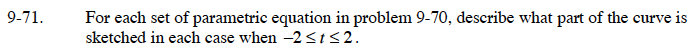### Home > CALC > Chapter Ch9 > Lesson 9.2.2 > Problem9-71

9-71.The curve will start at (cos(–2), sin(–2)), pass through (cos(0), sin(0)), and end at (cos(2), sin(2)).
Evaluate and graph these points.

The curve will start at (cos(–4), sin(–4)), pass through (cos(0), sin(0)), and end at (cos(4), sin(4)).
Evaluate and graph these points.